# 3.7 Inverse functions  (Page 8/9)

 Page 8 / 9

$f\left(x\right)=-3x+5$

one-to-one

$f\left(x\right)=|x-3|$

For the following exercises, use the vertical line test to determine if the relation whose graph is provided is a function.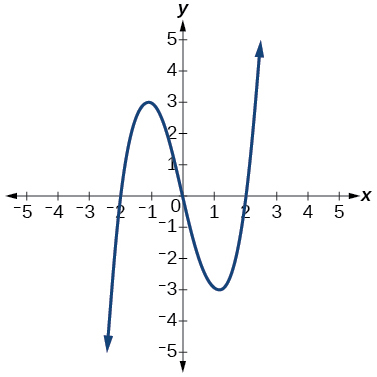function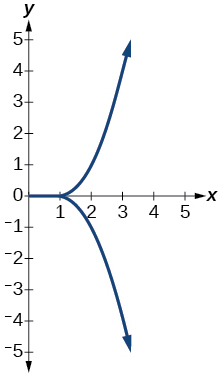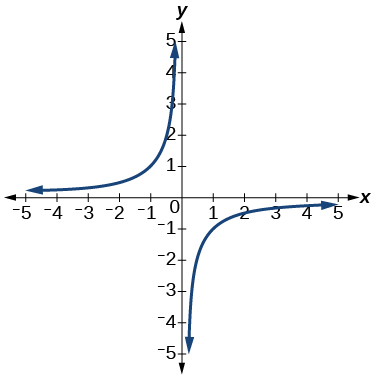function

For the following exercises, graph the functions.

$f\left(x\right)=|x+1|$

$f\left(x\right)={x}^{2}-2$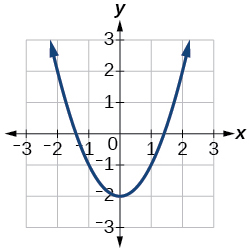For the following exercises, use [link] to approximate the values.

$f\left(2\right)$

$f\left(-2\right)$

$2$

If $\text{\hspace{0.17em}}f\left(x\right)=-2,\text{\hspace{0.17em}}$ then solve for $\text{\hspace{0.17em}}x.$

If $\text{\hspace{0.17em}}f\left(x\right)=1,\text{\hspace{0.17em}}$ then solve for $\text{\hspace{0.17em}}x.$

or

For the following exercises, use the function $\text{\hspace{0.17em}}h\left(t\right)=-16{t}^{2}+80t\text{\hspace{0.17em}}$ to find the values in simplest form.

$\frac{h\left(2\right)-h\left(1\right)}{2-1}$

$\frac{h\left(a\right)-h\left(1\right)}{a-1}$

$\frac{-64+80a-16{a}^{2}}{-1+a}=-16a+64$

## Domain and Range

For the following exercises, find the domain of each function, expressing answers using interval notation.

$f\left(x\right)=\frac{2}{3x+2}$

$f\left(x\right)=\frac{x-3}{{x}^{2}-4x-12}$

$\left(-\infty ,-2\right)\cup \left(-2,6\right)\cup \left(6,\infty \right)$

$f\left(x\right)=\frac{\sqrt{x-6}}{\sqrt{x-4}}$

Graph this piecewise function: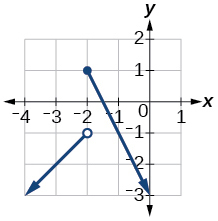## Rates of Change and Behavior of Graphs

For the following exercises, find the average rate of change of the functions from

$f\left(x\right)=4x-3$

$f\left(x\right)=10{x}^{2}+x$

$31$

$f\left(x\right)=-\frac{2}{{x}^{2}}$

For the following exercises, use the graphs to determine the intervals on which the functions are increasing, decreasing, or constant.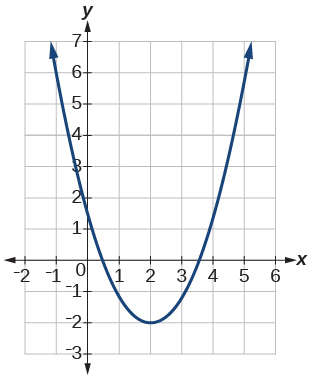increasing $\text{\hspace{0.17em}}\left(2,\infty \right);\text{\hspace{0.17em}}$ decreasing $\text{\hspace{0.17em}}\left(-\infty ,2\right)$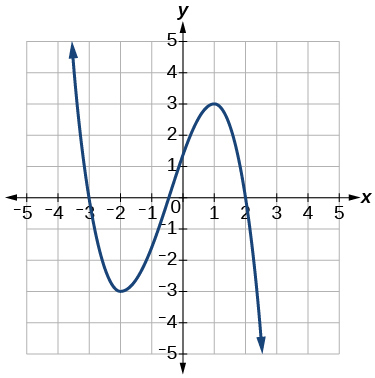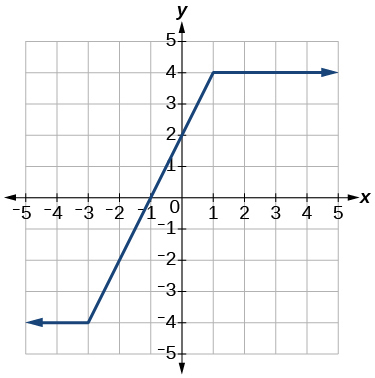increasing $\text{}\left(-3,1\right);\text{}$ constant $\text{\hspace{0.17em}}\left(-\infty ,-3\right)\cup \left(1,\infty \right)$

Find the local minimum of the function graphed in [link] .

Find the local extrema for the function graphed in [link] .

local minimum $\text{\hspace{0.17em}}\left(-2,-3\right);\text{\hspace{0.17em}}$ local maximum $\text{\hspace{0.17em}}\left(1,3\right)$

For the graph in [link] , the domain of the function is $\text{\hspace{0.17em}}\left[-3,3\right].$ The range is $\text{\hspace{0.17em}}\left[-10,10\right].\text{\hspace{0.17em}}$ Find the absolute minimum of the function on this interval.

Find the absolute maximum of the function graphed in [link] .

$\text{\hspace{0.17em}}\left(-1.8,10\right)\text{\hspace{0.17em}}$

## Composition of Functions

For the following exercises, find $\text{\hspace{0.17em}}\left(f\circ g\right)\left(x\right)\text{\hspace{0.17em}}$ and $\text{\hspace{0.17em}}\left(g\circ f\right)\left(x\right)\text{\hspace{0.17em}}$ for each pair of functions.

$f\left(x\right)=4-x,\text{\hspace{0.17em}}g\left(x\right)=-4x$

$f\left(x\right)=3x+2,\text{\hspace{0.17em}}g\left(x\right)=5-6x$

$\left(f\circ g\right)\left(x\right)=17-18x;\text{\hspace{0.17em}}\left(g\circ f\right)\left(x\right)=-7-18x$

$f\left(x\right)={x}^{2}+2x,\text{\hspace{0.17em}}g\left(x\right)=5x+1$

$\left(f\circ g\right)\left(x\right)=\sqrt{\frac{1}{x}+2};\text{\hspace{0.17em}}\left(g\circ f\right)\left(x\right)=\frac{1}{\sqrt{x+2}}$

For the following exercises, find $\text{\hspace{0.17em}}\left(f\circ g\right)\text{\hspace{0.17em}}$ and the domain for $\text{\hspace{0.17em}}\left(f\circ g\right)\left(x\right)\text{\hspace{0.17em}}$ for each pair of functions.

$\left(f\circ g\right)\left(x\right)=\frac{1}{\sqrt{x}},\text{\hspace{0.17em}}x>0$

For the following exercises, express each function $\text{\hspace{0.17em}}H\text{\hspace{0.17em}}$ as a composition of two functions $\text{\hspace{0.17em}}f\text{\hspace{0.17em}}$ and $\text{\hspace{0.17em}}g\text{\hspace{0.17em}}$ where $\text{\hspace{0.17em}}H\left(x\right)=\left(f\circ g\right)\left(x\right).$

$H\left(x\right)=\sqrt{\frac{2x-1}{3x+4}}$

sample: $\text{\hspace{0.17em}}g\left(x\right)=\frac{2x-1}{3x+4};\text{\hspace{0.17em}}f\left(x\right)=\sqrt{x}$

$H\left(x\right)=\frac{1}{{\left(3{x}^{2}-4\right)}^{-3}}$

## Transformation of Functions

For the following exercises, sketch a graph of the given function.

$f\left(x\right)={\left(x-3\right)}^{2}$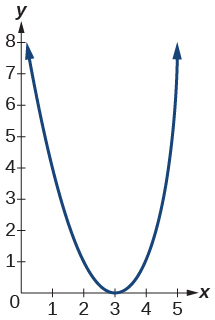$f\left(x\right)={\left(x+4\right)}^{3}$

$f\left(x\right)=\sqrt{x}+5$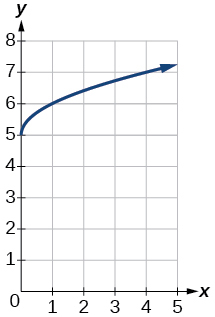$f\left(x\right)=-{x}^{3}$

$f\left(x\right)=\sqrt{-x}$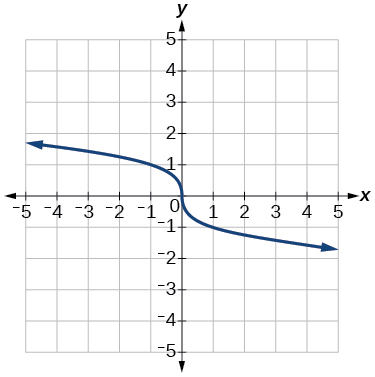$f\left(x\right)=5\sqrt{-x}-4$

$f\left(x\right)=4\left[|x-2|-6\right]$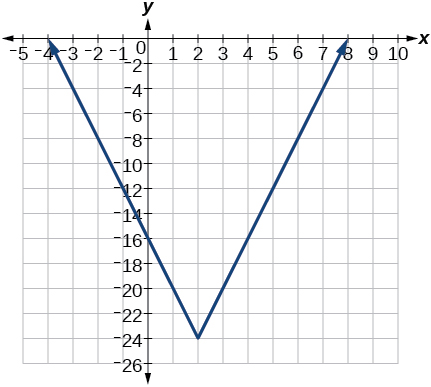$f\left(x\right)=-{\left(x+2\right)}^{2}-1$

For the following exercises, sketch the graph of the function $\text{\hspace{0.17em}}g\text{\hspace{0.17em}}$ if the graph of the function $\text{\hspace{0.17em}}f\text{\hspace{0.17em}}$ is shown in [link] .

$g\left(x\right)=f\left(x-1\right)$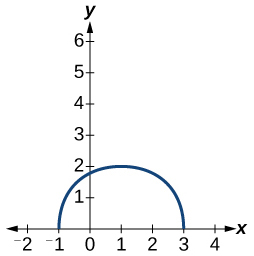$g\left(x\right)=3f\left(x\right)$

For the following exercises, write the equation for the standard function represented by each of the graphs below.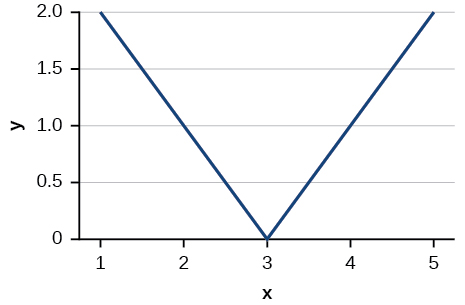$f\left(x\right)=|x-3|$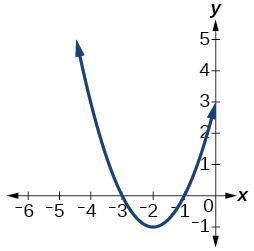For the following exercises, determine whether each function below is even, odd, or neither.

$f\left(x\right)=3{x}^{4}$

even

$g\left(x\right)=\sqrt{x}$

$h\left(x\right)=\frac{1}{x}+3x$

odd

For the following exercises, analyze the graph and determine whether the graphed function is even, odd, or neither.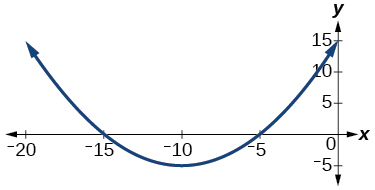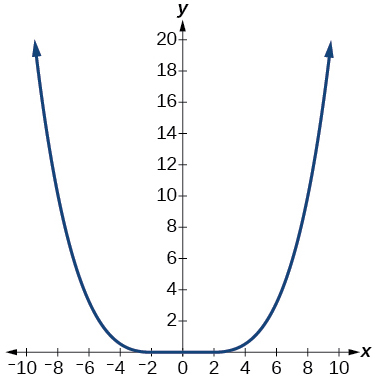even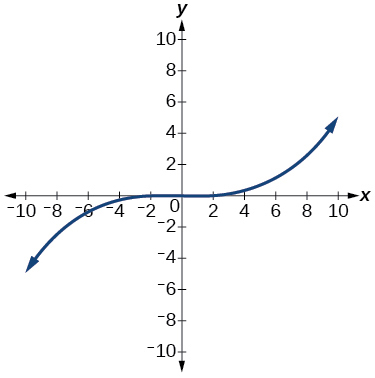## Absolute Value Functions

For the following exercises, write an equation for the transformation of $\text{\hspace{0.17em}}f\left(x\right)=|x|.$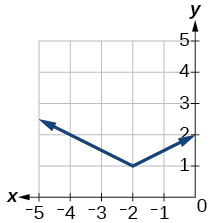$f\left(x\right)=\frac{1}{2}|x+2|+1$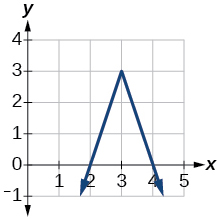$f\left(x\right)=-3|x-3|+3$

For the following exercises, graph the absolute value function.

$f\left(x\right)=|x-5|$

$f\left(x\right)=-|x-3|$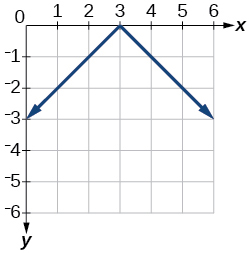$f\left(x\right)=|2x-4|$

## Inverse Functions

For the following exercises, find for each function.

$f\left(x\right)=9+10x$

$f\left(x\right)=\frac{x}{x+2}$

${f}^{-1}\left(x\right)=\frac{-2x}{x-1}$

For the following exercise, find a domain on which the function is one-to-one and non-decreasing. Write the domain in interval notation. Then find the inverse of restricted to that domain.

$f\left(x\right)={x}^{2}+1$

Given $f\left(x\right)={x}^{3}-5$ and $g\left(x\right)=\sqrt{x+5}:$

1. Find and $g\left(f\left(x\right)\right).$
2. What does the answer tell us about the relationship between $f\left(x\right)$ and $g\left(x\right)?$
1. and $g\left(f\left(x\right)\right)=x.$
2. This tells us that $f$ and $g$ are inverse functions

For the following exercises, use a graphing utility to determine whether each function is one-to-one.

$f\left(x\right)=\frac{1}{x}$

The function is one-to-one.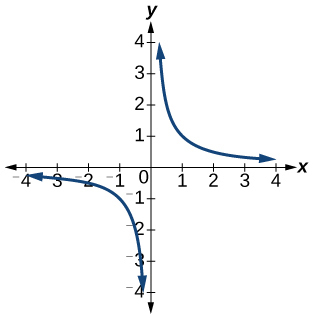$f\left(x\right)=-3{x}^{2}+x$

The function is not one-to-one.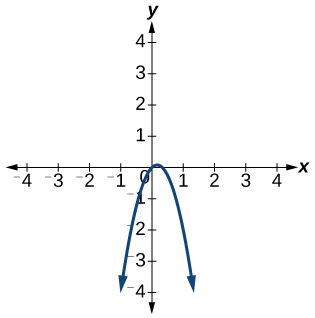If $f\left(5\right)=2,$ find ${f}^{-1}\left(2\right).$

$5$

If $f\left(1\right)=4,$ find ${f}^{-1}\left(4\right).$

## Practice test

For the following exercises, determine whether each of the following relations is a function.

$y=2x+8$

The relation is a function.

$\left\{\left(2,1\right),\left(3,2\right),\left(-1,1\right),\left(0,-2\right)\right\}$

For the following exercises, evaluate the function $\text{\hspace{0.17em}}f\left(x\right)=-3{x}^{2}+2x\text{\hspace{0.17em}}$ at the given input.

$f\left(-2\right)$

−16

$\text{\hspace{0.17em}}f\left(a\right)\text{\hspace{0.17em}}$

Show that the function $\text{\hspace{0.17em}}f\left(x\right)=-2{\left(x-1\right)}^{2}+3\text{\hspace{0.17em}}$ is not one-to-one.

The graph is a parabola and the graph fails the horizontal line test.

Write the domain of the function $\text{\hspace{0.17em}}f\left(x\right)=\sqrt{3-x}\text{\hspace{0.17em}}$ in interval notation.

Given $\text{\hspace{0.17em}}f\left(x\right)=2{x}^{2}-5x,\text{\hspace{0.17em}}$ find $f\left(a+1\right)-f\left(1\right)\text{\hspace{0.17em}}$ in simplest form.

$2{a}^{2}-a$

Graph the function

Find the average rate of change of the function $\text{\hspace{0.17em}}f\left(x\right)=3-2{x}^{2}+x\text{\hspace{0.17em}}$ by finding $\text{\hspace{0.17em}}\frac{f\left(b\right)-f\left(a\right)}{b-a}\text{\hspace{0.17em}}$ in simplest form.

$-2\left(a+b\right)+1$

For the following exercises, use the functions to find the composite functions.

$\left(g\circ f\right)\left(x\right)$

$\left(g\circ f\right)\left(1\right)$

$\sqrt{2}$

Express $\text{\hspace{0.17em}}H\left(x\right)=\sqrt{5{x}^{2}-3x}\text{\hspace{0.17em}}$ as a composition of two functions, $\text{\hspace{0.17em}}f\text{\hspace{0.17em}}$ and $\text{\hspace{0.17em}}g,\text{\hspace{0.17em}}$ where $\text{\hspace{0.17em}}\left(f\circ g\right)\left(x\right)=H\left(x\right).$

For the following exercises, graph the functions by translating, stretching, and/or compressing a toolkit function.

$f\left(x\right)=\sqrt{x+6}-1$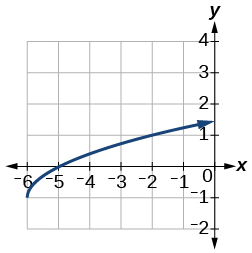$f\left(x\right)=\frac{1}{x+2}-1$

For the following exercises, determine whether the functions are even, odd, or neither.

$f\left(x\right)=-\frac{5}{{x}^{2}}+9{x}^{6}$

$\text{even}$

$f\left(x\right)=-\frac{5}{{x}^{3}}+9{x}^{5}$

$f\left(x\right)=\frac{1}{x}$

$\text{odd}$

Graph the absolute value function $\text{\hspace{0.17em}}f\left(x\right)=-2|x-1|+3.$

For the following exercises, find the inverse of the function.

$f\left(x\right)=3x-5$

${f}^{-1}\left(x\right)=\frac{x+5}{3}$

$f\left(x\right)=\frac{4}{x+7}$

For the following exercises, use the graph of $\text{\hspace{0.17em}}g\text{\hspace{0.17em}}$ shown in [link] .

On what intervals is the function increasing?

On what intervals is the function decreasing?

Approximate the local minimum of the function. Express the answer as an ordered pair.

$\left(1.1,-0.9\right)$

Approximate the local maximum of the function. Express the answer as an ordered pair.

For the following exercises, use the graph of the piecewise function shown in [link] .

Find $\text{\hspace{0.17em}}f\left(2\right).$

$f\left(2\right)=2$

Find $\text{\hspace{0.17em}}f\left(-2\right).$

Write an equation for the piecewise function.

$f\left(x\right)=\left\{\begin{array}{c}|x|\text{\hspace{0.17em}}\text{\hspace{0.17em}}\text{\hspace{0.17em}}\text{if}\text{\hspace{0.17em}}\text{\hspace{0.17em}}x\le 2\\ 3\text{\hspace{0.17em}}\text{\hspace{0.17em}}\text{\hspace{0.17em}}\text{\hspace{0.17em}}\text{\hspace{0.17em}}\text{if}\text{\hspace{0.17em}}\text{\hspace{0.17em}}x>2\end{array}$

For the following exercises, use the values listed in [link] .

$x$ $F\left(x\right)$
0 1
1 3
2 5
3 7
4 9
5 11
6 13
7 15
8 17

Find $\text{\hspace{0.17em}}F\left(6\right).$

Solve the equation $\text{\hspace{0.17em}}F\left(x\right)=5.$

$x=2$

Is the graph increasing or decreasing on its domain?

Is the function represented by the graph one-to-one?

yes

Find $\text{\hspace{0.17em}}{F}^{-1}\left(15\right).$

Given $\text{\hspace{0.17em}}f\left(x\right)=-2x+11,\text{\hspace{0.17em}}$ find $\text{\hspace{0.17em}}{f}^{-1}\left(x\right).$

${f}^{-1}\left(x\right)=-\frac{x-11}{2}$

#### Questions & Answers

write down the polynomial function with root 1/3,2,-3 with solution
if A and B are subspaces of V prove that (A+B)/B=A/(A-B)
write down the value of each of the following in surd form a)cos(-65°) b)sin(-180°)c)tan(225°)d)tan(135°)
Prove that (sinA/1-cosA - 1-cosA/sinA) (cosA/1-sinA - 1-sinA/cosA) = 4
what is the answer to dividing negative index
In a triangle ABC prove that. (b+c)cosA+(c+a)cosB+(a+b)cisC=a+b+c.
give me the waec 2019 questions
the polar co-ordinate of the point (-1, -1)
prove the identites sin x ( 1+ tan x )+ cos x ( 1+ cot x )= sec x + cosec x
tanh`(x-iy) =A+iB, find A and B
B=Ai-itan(hx-hiy)
Rukmini
what is the addition of 101011 with 101010
If those numbers are binary, it's 1010101. If they are base 10, it's 202021.
Jack
extra power 4 minus 5 x cube + 7 x square minus 5 x + 1 equal to zero
the gradient function of a curve is 2x+4 and the curve passes through point (1,4) find the equation of the curve
1+cos²A/cos²A=2cosec²A-1
test for convergence the series 1+x/2+2!/9x3ByByByBy